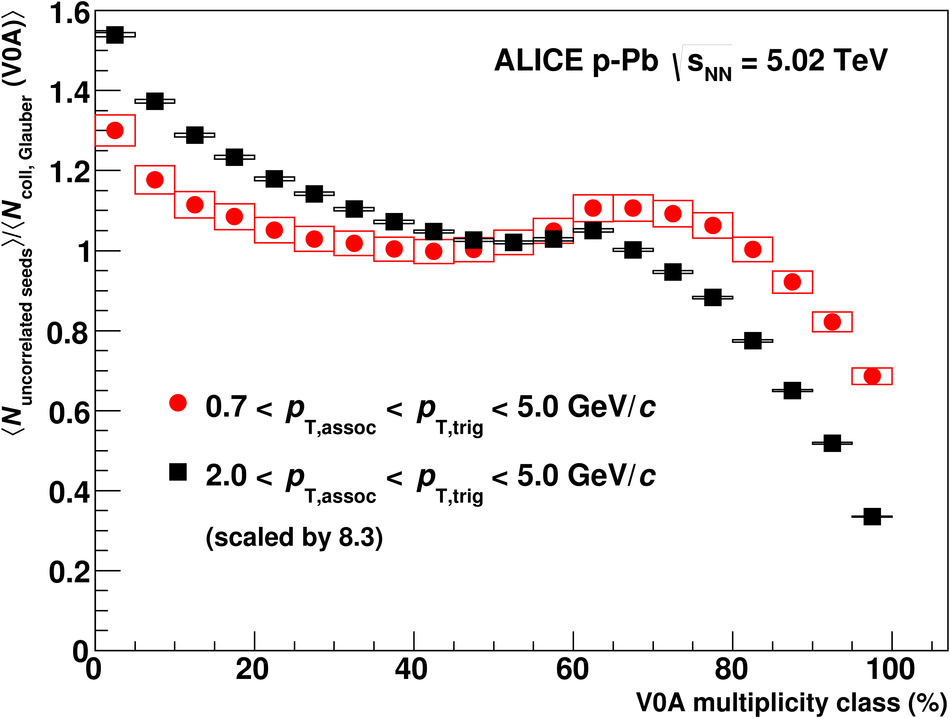# Figure 6

 Ratio between uncorrelated seeds and $N_{\rm coll}$ estimated within the Glauber model as a function of V0A multiplicity class. Statistical (lines) and systematic uncertainties (boxes) are shown, even though the statistical ones are smaller than the symbol size. To aid the comparison, the higher $\pt$ range has been scaled by a factor 8.3 to agree with the lower $\pt$ range in the 50-55% multiplicity class